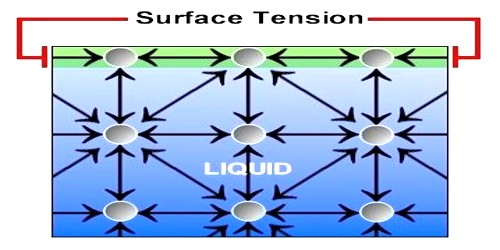# Which Factors are Influencing the Surface Tension of a Liquid?

Factors influencing the surface tension of a liquid:

The surface tension of a liquid depends on the following factors:

(i) Contamination: If the liquid surface gets contaminated by oily or greasy substances its surface tension in general decreases.

(ii) Presence of dissolved substances: If a substance is dissolved in a liquid the surface tension of that liquid is changed. If inorganic substances are dissolved in liquid surface tension of the liquid increases but if organic substances is dissolved the surface tension decreases.

(iii) Temperature: Surface tension of liquid is largely dependent on temperature. Generally the surface tension decreases with the increase of temperature and increases with the decrease of temperature. In surface tension a critical temperature disappears i.e. is absent or zero. Exceptions are noticed only in case of melted copper and cadmium. Within a small range of temperature, relation between surface tension Tt and temperature t, is –

Tt = T0 (1 – at)

here, Tt = Surface tension at temperature t0C, T0 =  Surface tension at 00C and α is the temperature co-efficient of surface tension.

(iv) Medium above the liquid: Surface tension of a liquid depends on the nature of the medium above free surface. For example- surface tension of water is 70 x10-3 Nm-1, when vapour is above the water surface. On the other hand it is 72 x 10-1 Nm-1, when air is in contact with water.

(vi) Electrification: When a liquid is electrified, surface tension decreases. Since external force acts on the liquid surface due to electrification, so area of the liquid surface increases which acts against contraction phenomenon of surface tension. Hence, it also decreases.